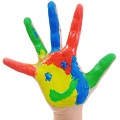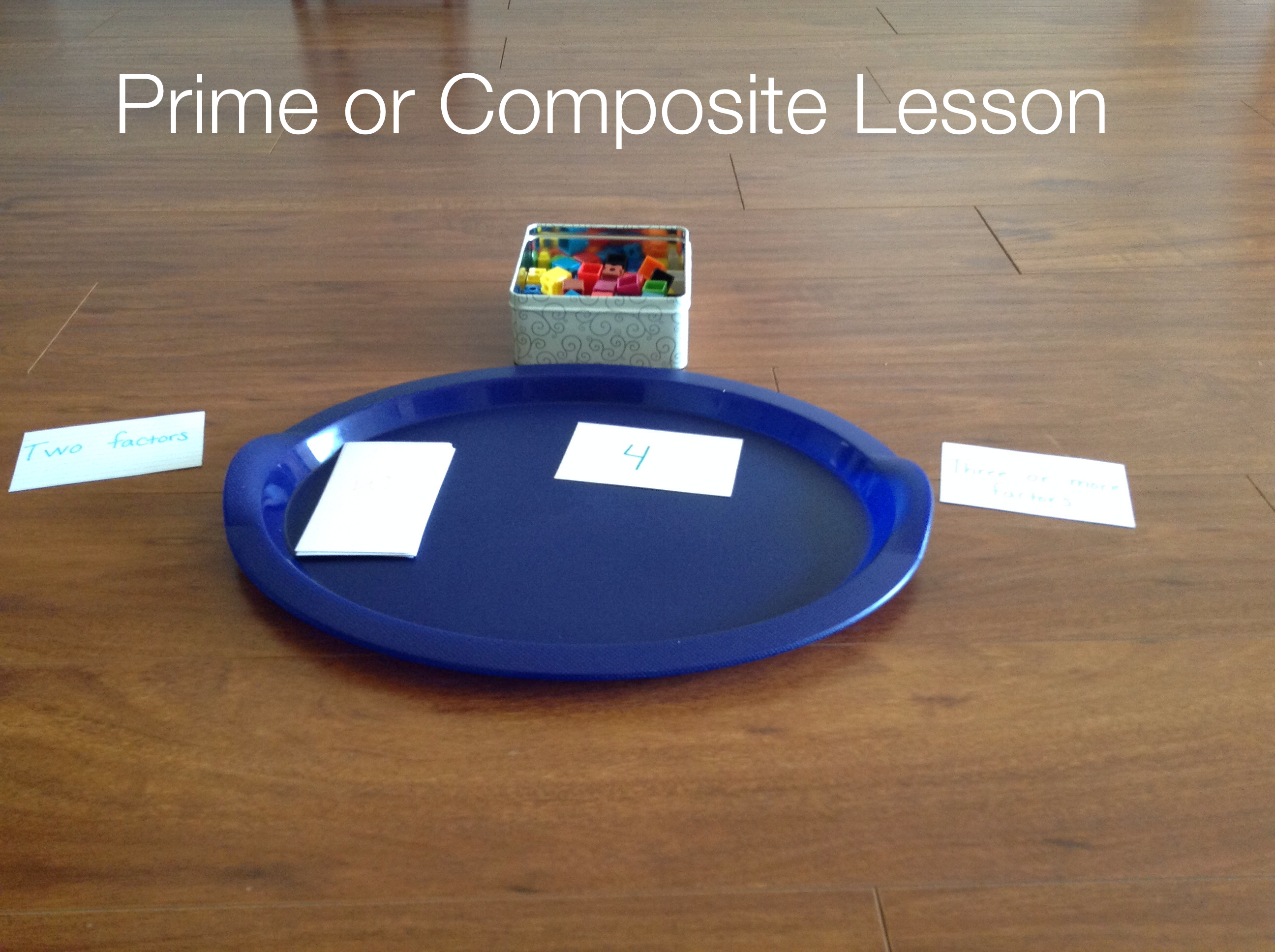# Hands-on Math: Teaching Prime and Composite

If you’ve read my math posts before you know that I strongly encourage parents to make math hands-on as much as possible.  Everything we learn is rooted in concrete experiences. Therefore,  the more hands-on experience we give our children, the stronger their mathematical foundation will be!  Even older kids can benefit from hands-on math experiences.  In this post I will show you how your child can practice the concept of prime and composite numbers in a concrete way.

### Prime and Composite Numbers

Prime numbers are simply numbers that have only two factors, one and itself.  For example, 3 is prime.  The only factors of 3 are 3 and 1.  Composite numbers have three or more factors. For example, 6 is composite. The number 6 can also be divided into two groups of three or three groups of two. It’s factors are 1, 2, 3, and 6.

Teaching Tip: It is important to think about the background knowledge needed for a lesson.  In this lesson, I am assuming the child has a good understanding of multiplication and division.  The student should also understand what a factor is.  If the child does not know what a factor is, I would teach that first.

Preparation:

• First, prepare index cards with various numbers on them.  Have a mixture of prime and composite numbers.  Don’t make the numbers too large.  You want them to be manageable.  I made cards with the numbers 2 through 14.
• Gather your linking cubes (or other small similar items) and put them in a bowl.
• Create two index cards labeled, “Two factors” and “Three or more factors”
• Create two index cards labeled, “Prime” and “Composite”

Set up tray like this (or what works for you!):

Activity:

1. To demonstrate the activity, I put the number 4 face up.  I wanted to use a composite number first.  I had a prime number next in line (3).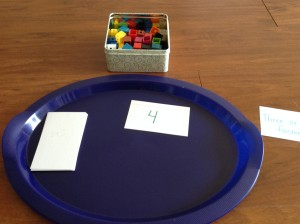2.  Looking at the four, ask the child to take out the corresponding number of cubes.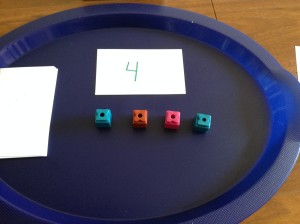3.  Next, ask the child if the number (4) can be divided into equal groups. Have him or her actually divide them into groups using the cubes.  You should have two groups of two.  Remind the child that two is a factor of four.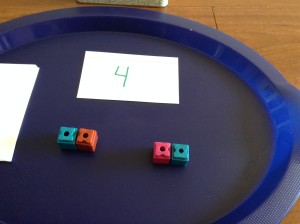4.  Ask your child to figure out all the factors of the number 4, demonstrating the factors by grouping the cubes. Have your child list the factors on the card.

5. Have your child note how many factors the number 4 has.  Tell your child that we are going to sort the numbers based on how many factors they have. If it has two factors, place it in the “Two factors” group.  If it has three or more factors put it in the “Three or more factors” group.

6. Using a prime number next (in this case 3),  have the child take out the corresponding numbers of cubes. Ask the child if he or she can divide three into any other equal groupings.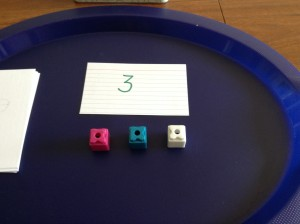7. Have the child write the factors on the card and put it into it’s proper group.

8. Continue this until the child has done the procedure with all of the cards.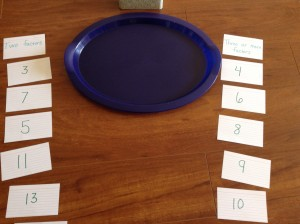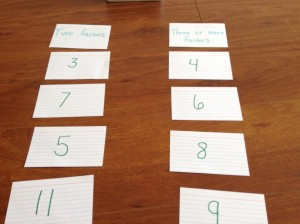9.  Once all the cards have been sorted, draw your child’s attention to the two groups.  Tell your child that numbers that have only two factors- one and itself are called prime numbers.  Place the index card “Prime” over the card labeled “Two factors”.  Numbers that have three of more factors are called composite.  Place the index card labeled “Composite” over the card labeled, “Three or more factors”.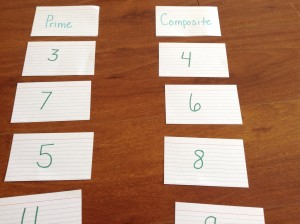10. To see if your child understands, give him or her a number and ask if the number is prime or composite.  For example, is 16 prime or composite?  He or she may be able to answer right away or may need to use the cubes. Ask the child how s/he knows this.  The child should be able to articulate that sixteen has more than three factors, possibly even listing the factors.

11. If the child is confused, you may need to try the exercise again with a little more support. Or, possibly, the child may need to work on a background skill first, such as factors.

I hope you enjoy this activity together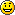For supporting literature check out (affiliate link):